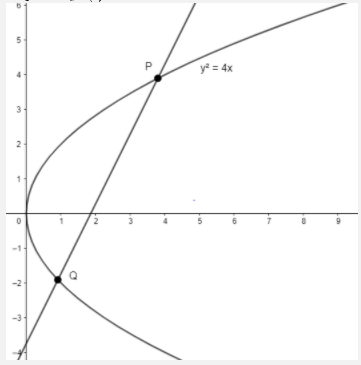Courses
Courses for Kids
Free study material
Offline Centres
MoreLast updated date: 28th Nov 2023
Total views: 382.5k
Views today: 5.82k

# The locus of a point which divides a chord of slope 2 of the parabola ${{y}^{2}}=4x$ internally in the ratio 1:2 is(a) ${{\left( y+\dfrac{4}{9} \right)}^{2}}=\dfrac{4}{9}\left( x-\dfrac{2}{9} \right)$(b) ${{\left( y-\dfrac{8}{9} \right)}^{2}}=\dfrac{4}{9}\left( x-\dfrac{2}{9} \right)$(c) ${{\left( y-\dfrac{8}{9} \right)}^{2}}=\dfrac{4}{9}\left( x+\dfrac{2}{9} \right)$(d) ${{\left( y+\dfrac{8}{9} \right)}^{2}}=\dfrac{4}{9}\left( x+\dfrac{2}{9} \right)$Verified
382.5k+ views
Hint: To find the locus of point which divides a chord of given slope of the parabola internally in the ratio 1:2, write the equation of chord joining any two points of the parabola and then find the point which divides these two points on the parabola internally in the ratio 1:2.

We have a parabola ${{y}^{2}}=4x$.We have to find the locus of point on a chord of slope 2 which divides the chord internally in the ratio 1:2.
Let’s assume that there are two points on parabola $P\left( {{t}_{1}} \right)$and$Q\left( {{t}_{2}} \right)$.
The equation of chord of the parabola ${{y}^{2}}=4ax$ joining these two points $P\left( {{t}_{1}} \right)$ and $Q\left( {{t}_{2}} \right)$is$y\left( {{t}_{1}}+{{t}_{2}} \right)=2x+2a{{t}_{1}}{{t}_{2}}$.
We observe that $a=1$ in our case.
By substituting the value, we have $y\left( {{t}_{1}}+{{t}_{2}} \right)=2x+2{{t}_{1}}{{t}_{2}}$
Dividing the equation by $\left( {{t}_{1}}+{{t}_{2}} \right)$, we get
$y=\dfrac{2x}{{{t}_{1}}+{{t}_{2}}}+\dfrac{2{{t}_{1}}{{t}_{2}}}{{{t}_{1}}+{{t}_{2}}}$
We know the slope of this chord is 2, thus, $\dfrac{2}{{{t}_{1}}+{{t}_{2}}}=2$
$\Rightarrow {{t}_{1}}+{{t}_{2}}=1$
$\Rightarrow {{t}_{2}}=1-{{t}_{1}}$ $-\left( 1 \right)$Now, we know that the formula of point which internally divides two points $\left( a,b \right)$and $\left( c,d \right)$in the ratio $m:n$ is$\left( \dfrac{am+cn}{m+n},\dfrac{bm+dn}{m+n} \right)$.
If a point divides any two points externally, then we replace + by - in the above formula.
So, let’s assume that the locus of our point which divides the chord with end points$\left( t_{1}^{2},2{{t}_{1}} \right)$and $\left( t_{2}^{2},2{{t}_{2}} \right)$ in the ratio $1:2$ is $\left( x,y \right)$
$\Rightarrow \left( x,y \right)=\left( \dfrac{t_{2}^{2}+2t_{1}^{2}}{3},\dfrac{2{{t}_{2}}+4{{t}_{1}}}{3} \right)$
$\Rightarrow x=\dfrac{t_{2}^{2}+2t_{1}^{2}}{3}$ and $y=\dfrac{2{{t}_{2}}+4{{t}_{1}}}{3}$
Substituting using equation $\left( 1 \right)$,we get
$\Rightarrow x=\dfrac{{{\left( 1-{{t}_{1}} \right)}^{2}}+2t_{1}^{2}}{3}=\dfrac{3t_{1}^{2}+1-2{{t}_{1}}}{3}$$=t_{1}^{2}+\dfrac{1}{3}-\dfrac{2{{t}_{1}}}{3}$ $\left( 2 \right)$
$\Rightarrow y=\dfrac{2\left( 1-{{t}_{1}} \right)+4{{t}_{1}}}{3}=\dfrac{2+2{{t}_{1}}}{3}$
Solving ${{t}_{1}}$ in terms of $y$ by multiplying the equation by 3, subtracting 2 and then dividing by 2 on both sides, we get $\dfrac{3y-2}{2}={{t}_{1}}$ $\left( 3 \right)$
Substituting using equation $\left( 3 \right)$in equation$\left( 2 \right)$, we get$x={{\left( \dfrac{3y}{2}-1 \right)}^{2}}+\dfrac{1}{3}-\left( \dfrac{3y-2}{3} \right)=\dfrac{9{{y}^{2}}}{4}+1-3y+\dfrac{1}{3}-y+\dfrac{2}{3}=\dfrac{9{{y}^{2}}}{4}-4y+2$.
Multiplying the equation by $\dfrac{4}{9}$, we get $\dfrac{4x}{9}={{y}^{2}}-\dfrac{16y}{9}+\dfrac{8}{9}$
Subtracting $\dfrac{8}{81}$from both side of the equation, we get $\dfrac{4x}{9}-\dfrac{8}{81}={{y}^{2}}-\dfrac{16y}{9}+\dfrac{64}{81}$
Rearranging the terms, we get $\dfrac{4}{9}\left( x-\dfrac{2}{9} \right)={{\left( y-\dfrac{8}{9} \right)}^{2}}$
Hence, the correct answer is ${{\left( y-\dfrac{8}{9} \right)}^{2}}=\dfrac{4}{9}\left( x-\dfrac{2}{9} \right)$
Option (B) is the correct answer.
Note: We can also write the equation of chord in terms of a variable slope and then use it to find the locus of point which divides the chord in the ratio 1:2.